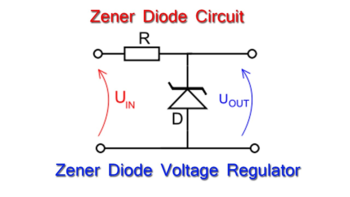# Zener Diode as Voltage Regulator1. VOLTAGE REGULATOR.

=> it is any device that outputs a constant input voltage for any input voltage.

voltage regulator is generally used to limit the voltage across any load that can be damaged by high voltage.

2. CHARACTER OF ZENER DIODE

1. under forward biasing the zener diode act just like a P-n junction diode

2. when it is reverse biased then it shows specific characters ;

-when the voltage is below the zener brealdown voltage then almost no current flows through it. so it acts like an open circuit

-when voltage is above the zener breakdown voltage then very large current flows through it, so it acts as a short-circuit

the breakdown voltage is sharp and constant for any zener diode.

3. ZENER DIODE AS VOLTAGE REGULATOR

Principle:  zener diode is connected in parallel   with a load in reverse bias so that the potential drop across the load remains equal to the zener breakdown voltage and the excess voltage is dropped across the series resistance.Setup;

CIRCUIT ARRANGEMENT

1. the load (Rl) is connected in series with the power source in series,

2, the diode should be connected in parallel with the load. the diode must be reverse biased so that large current can flow through the diode when excess voltage is supplied

3. there is a resistance connected in series across which the excess voltage is dropped.

WORKING

1. when input voltage is less than required voltage  Vz (zener voltage), then zener breakdown doesnt occour and no current flows through the diode.

ie Iz = 0, so all current flows through the load

2. when the input voltage is above the zener voltage, then

total current Iz + I2 increases.

here, Iz can increase to any value so I2 doesnt increase.

the Vz remains constant ie voltage across the load doesnt increase

and the potential drop across the series resistance increases.